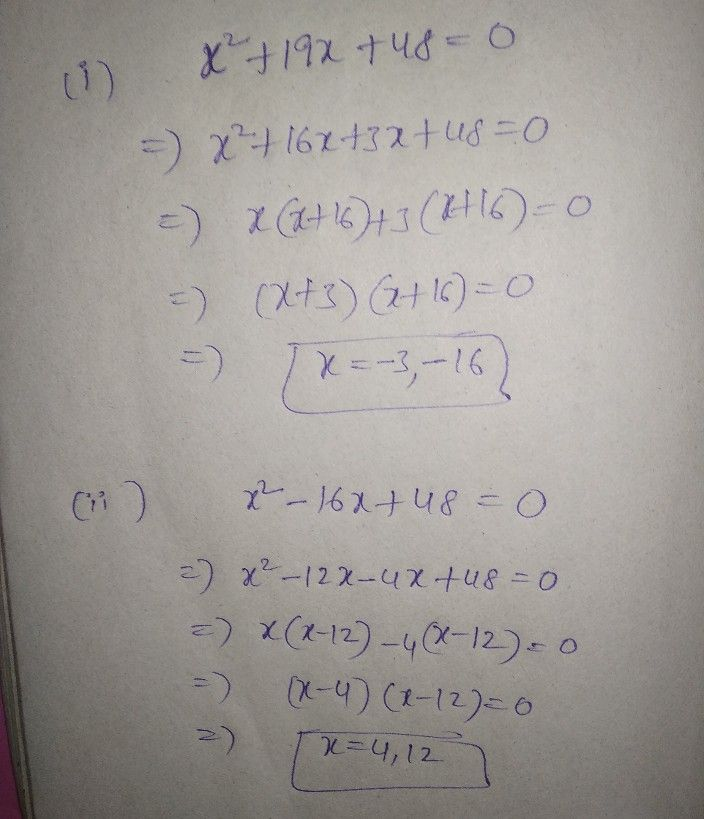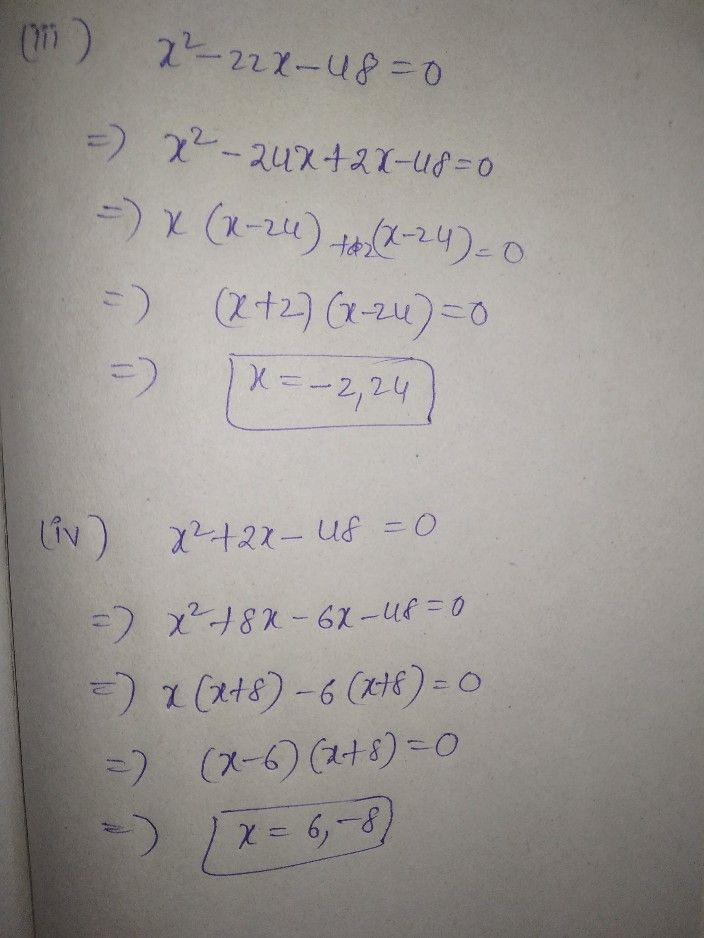Symbol
Problem$1\right)$ $x^{2}$ $+19x$ $+18$ $Q\right)$ $x^{2}$ $-16x+48$ $3\right)$ $x^{2}$ $-αdx$ $-48$ $y\right)$ $x^{2}$ $+dx$ $-48$